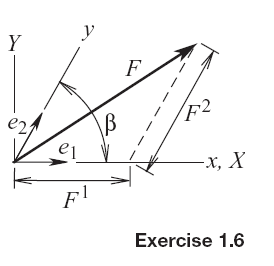### Create an Account

Already have account?

### Forgot Your Password ?

Home / Questions / An affine coordinate system has unit vectors that are not mutually orthogonal The x and y ...

# An affine coordinate system has unit vectors that are not mutually orthogonal The x and y axes in the sketch constitute such a system for a planar situation A vector may be represented in terms of

An affine coordinate system has unit vectors that are not mutually orthogonal. The and axes in the sketch constitute such a system for a planar situation. A vector may be represented in terms of its contravariant components relative to xy by constructing lines parallel to the respective coordinate axes. Such a set of components are F1 and F2 for the force vector in the sketch. The unit vectors parallel to the and axes are1 and2This force can also be represented in term of its Fand Fcomponents relative to the Cartesian XY coordinate system whose axis is coincident with x. Given that FX = 500 N, FY = 350 N, and β = 65°use vector algebra to determine F1 and F2.Jun 18 2020 View more View Less

#### Answer (Solved)Subscribe To Get Solution